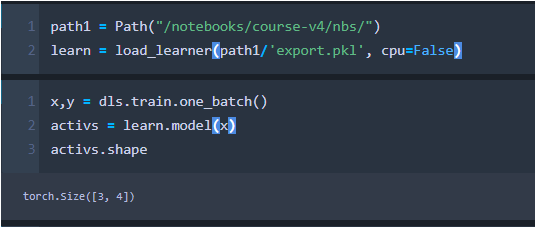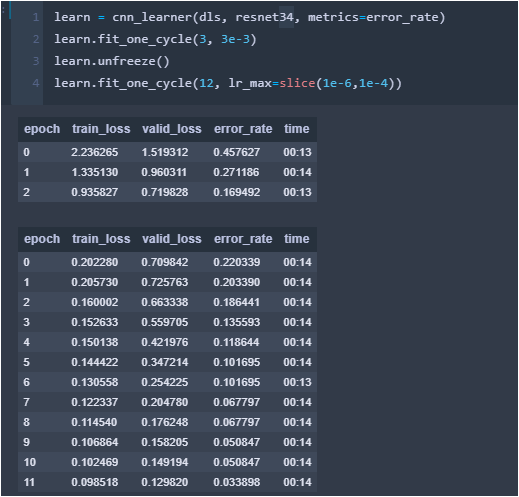# Importing Previous Models

I exported successully one of my model trained with

Resnet34 for 4 Categories images Resize to 224

Now, I would like to start the training of the Multicategory from my previous model, mentioned above.

I loaded in this way and then
`learn = load_learner(path/'export.pkl')`

I ran this

``````x,y = dls.train.one_batch()
activs = learn.model(x)
activs.shape
``````

And I got

`RuntimeError: Input type (torch.cuda.FloatTensor) and weight type (torch.FloatTensor) should be the same`

``````RuntimeError                              Traceback (most recent call last)
<ipython-input-19-7b26555ca519> in <module>
1 x,y = dls.train.one_batch()
----> 2 activs = learn.model(x)
3 activs.shape

/opt/conda/envs/fastai/lib/python3.7/site-packages/torch/nn/modules/module.py in __call__(self, *input, **kwargs)
530             result = self._slow_forward(*input, **kwargs)
531         else:
--> 532             result = self.forward(*input, **kwargs)
533         for hook in self._forward_hooks.values():
534             hook_result = hook(self, input, result)

/opt/conda/envs/fastai/lib/python3.7/site-packages/torch/nn/modules/container.py in forward(self, input)
98     def forward(self, input):
99         for module in self:
--> 100             input = module(input)
101         return input
102

/opt/conda/envs/fastai/lib/python3.7/site-packages/torch/nn/modules/module.py in __call__(self, *input, **kwargs)
530             result = self._slow_forward(*input, **kwargs)
531         else:
--> 532             result = self.forward(*input, **kwargs)
533         for hook in self._forward_hooks.values():
534             hook_result = hook(self, input, result)

/opt/conda/envs/fastai/lib/python3.7/site-packages/torch/nn/modules/container.py in forward(self, input)
98     def forward(self, input):
99         for module in self:
--> 100             input = module(input)
101         return input
102

/opt/conda/envs/fastai/lib/python3.7/site-packages/torch/nn/modules/module.py in __call__(self, *input, **kwargs)
530             result = self._slow_forward(*input, **kwargs)
531         else:
--> 532             result = self.forward(*input, **kwargs)
533         for hook in self._forward_hooks.values():
534             hook_result = hook(self, input, result)

/opt/conda/envs/fastai/lib/python3.7/site-packages/torch/nn/modules/conv.py in forward(self, input)
343
344     def forward(self, input):
--> 345         return self.conv2d_forward(input, self.weight)
346
347 class Conv3d(_ConvNd):

/opt/conda/envs/fastai/lib/python3.7/site-packages/torch/nn/modules/conv.py in conv2d_forward(self, input, weight)
340                             _pair(0), self.dilation, self.groups)
341         return F.conv2d(input, weight, self.bias, self.stride,
--> 342                         self.padding, self.dilation, self.groups)
343
344     def forward(self, input):

RuntimeError: Input type (torch.cuda.FloatTensor) and weight type (torch.FloatTensor) should be the same
``````

From my understanding it seems that the model that I am importing is ready for the CPU, but I am training on the GPU, so I can I integrate the two

You need to change your models’ device, or your input data’s device. load_learner defaults to CPU, pass cpu=False to load your model on the GPU (what I think you’re after)

2 Likes

Awesome Zach, like always, let me try.
It workedThank you so much

It is great to share this journey here.

After this first step, I am running into my second Issue:

I have this issue

`Target size (torch.Size([3, 5])) must be the same as input size (torch.Size([3, 4]))`

``````---------------------------------------------------------------------------
ValueError                                Traceback (most recent call last)
<ipython-input-26-38b1530800fe> in <module>
1 loss_func = nn.BCEWithLogitsLoss()
----> 2 loss = loss_func(activs, y)
3 loss

/opt/conda/envs/fastai/lib/python3.7/site-packages/torch/nn/modules/module.py in __call__(self, *input, **kwargs)
530             result = self._slow_forward(*input, **kwargs)
531         else:
--> 532             result = self.forward(*input, **kwargs)
533         for hook in self._forward_hooks.values():
534             hook_result = hook(self, input, result)

/opt/conda/envs/fastai/lib/python3.7/site-packages/torch/nn/modules/loss.py in forward(self, input, target)
599                                                   self.weight,
600                                                   pos_weight=self.pos_weight,
--> 601                                                   reduction=self.reduction)
602
603

/opt/conda/envs/fastai/lib/python3.7/site-packages/torch/nn/functional.py in binary_cross_entropy_with_logits(input, target, weight, size_average, reduce, reduction, pos_weight)
2122
2123     if not (target.size() == input.size()):
-> 2124         raise ValueError("Target size ({}) must be the same as input size ({})".format(target.size(), input.size()))
2125
2126     return torch.binary_cross_entropy_with_logits(input, target, weight, pos_weight, reduction_enum)
``````

This should be caused by the fact that I have 4 category in the previous model that I trained and in this one I have 5 categories.

1. How can I check the categories? there should be a “vocab” function
2. Not sure also why they are 5, even if in the Multicategory I placed 4.

dls.vocab I believe. You can also do dls.c to get the # of them

1 Like

Awesome Zack, it was counting the " ", space in my excel. Great catch. Thank you so much.

1 Like

Last step,
at the end when I am building the final learner:

learn = cnn_learner(dls, model, metrics=partial(accuracy_multi, thresh=0.2))
learn.fine_tune(3, base_lr=3e-3, freeze_epochs=4)

I used model and that model is the one that I load from my previous training:
model = load_learner(path1/‘export.pkl’, cpu=False)

``````---------------------------------------------------------------------------
TypeError                                 Traceback (most recent call last)
<ipython-input-69-cb015ccf8de5> in <module>
----> 1 learn = cnn_learner(dls, model, metrics=partial(accuracy_multi, thresh=0.2))
2 learn.fine_tune(3, base_lr=3e-3, freeze_epochs=4)

/opt/conda/envs/fastai/lib/python3.7/site-packages/fastai2/vision/learner.py in cnn_learner(dls, arch, loss_func, pretrained, cut, splitter, y_range, config, n_out, normalize, **kwargs)
173     if normalize: _add_norm(dls, meta, pretrained)
174     if y_range is None and 'y_range' in config: y_range = config.pop('y_range')
--> 175     model = create_cnn_model(arch, n_out, ifnone(cut, meta['cut']), pretrained, y_range=y_range, **config)
176     learn = Learner(dls, model, loss_func=loss_func, splitter=ifnone(splitter, meta['split']), **kwargs)
177     if pretrained: learn.freeze()

/opt/conda/envs/fastai/lib/python3.7/site-packages/fastai2/vision/learner.py in create_cnn_model(arch, n_out, cut, pretrained, n_in, init, custom_head, concat_pool, **kwargs)
99                      concat_pool=True, **kwargs):
100     "Create custom convnet architecture using `arch`, `n_in` and `n_out`"
--> 101     body = create_body(arch, n_in, pretrained, cut)
102     if custom_head is None:
103         nf = num_features_model(nn.Sequential(*body.children())) * (2 if concat_pool else 1)

/opt/conda/envs/fastai/lib/python3.7/site-packages/fastai2/vision/learner.py in create_body(arch, n_in, pretrained, cut)
64 def create_body(arch, n_in=3, pretrained=True, cut=None):
65     "Cut off the body of a typically pretrained `arch` as determined by `cut`"
---> 66     model = arch(pretrained=pretrained)
67     _update_first_layer(model, n_in, pretrained)
68     #cut = ifnone(cut, cnn_config(arch)['cut'])
``````

TypeError: call() got an unexpected keyword argument 'pretrained’

You shouldn’t use cnn_learner anymore then. It’s not designed for thisyou should make a Learner instead and make sure to pass in the loss function

(You can also do splitter=default_split and then call learn.freeze() and we just mimicked cnn_learner)

1 Like

Thank you so much Zack, I think I am getting closer, and I am learning a lot of new things which is great. Thanks

I was looking at the documentation:
https://docs.fast.ai/basic_train.html#Learner

`Learner` ( `data` : `DataBunch` , `model` : `Module` , `opt_func` : `Callable` = `'Adam'` , `loss_func` : `Callable` = `None` , `metrics` : `Collection` [ `Callable` ]= `None` , `true_wd` : `bool` = `True` , `bn_wd` : `bool` = `True` , `wd` : `Floats` = `0.01` , `train_bn` : `bool` = `True` , `path` : `str` = `None` , `model_dir` : `PathOrStr` = `'models'` , `callback_fns` : `Collection` [ `Callable` ]= `None` , `callbacks` : `Collection` [ `Callback` ]= `<factory>` , `layer_groups` : `ModuleList` = `None` , `add_time` : `bool` = `True` , `silent` : `bool` = `None` )

model:Module?

I create this learner
`learn = Learner(dls, model=model, loss_func = nn.BCEWithLogitsLoss(), metrics=partial(accuracy_multi, thresh=0.2), path=path)`

but I got this message

``````**---------------------------------------------------------------------------**
**AttributeError                            Traceback (most recent call last)**
**<ipython-input-73-62a02725519a> in <module>**
**----> 1 learn = Learner(dls, model=model, loss_func = nn.BCEWithLogitsLoss(), metrics=partial(accuracy_multi, thresh=0.2), path=path)**

**/opt/conda/envs/fastai/lib/python3.7/site-packages/fastai2/learner.py in __init__(self, dls, model, loss_func, opt_func, lr, splitter, cbs, metrics, path, model_dir, wd, wd_bn_bias, train_bn, moms)**
**     84         self.path = Path(path) if path is not None else getattr(dls, 'path', Path('.'))**
**     85         self.add_cbs([(cb() if isinstance(cb, type) else cb) for cb in L(defaults.callbacks)+L(cbs)])**
**---> 86         self.model.to(self.dls.device)**
**     87         if hasattr(self.model, 'reset'): self.model.reset()**
**     88         self.epoch,self.n_epoch,self.loss = 0,1,tensor(0.)**

**AttributeError: 'Learner' object has no attribute 'to'**
``````

Are there any reference or other examples to look at?

Probably I should have look at the
This documentation
https://dev.fast.ai/learner

`Learner` ( `dls` , `model` , `loss_func` = `None` , `opt_func` = `'Adam'` , `lr` = `0.001` , `splitter` = `'trainable_params'` , `cbs` = `None` , `metrics` = `None` , `path` = `None` , `model_dir` = `'models'` , `wd` = `None` , `wd_bn_bias` = `False` , `train_bn` = `True` , `moms` = `(0.95, 0.85, 0.95)` )

But still same issue: AttributeError: ‘Learner’ object has no attribute 'to’

this is a learner object, to get your model you will ahve to do learn.model

not sure if this will work but try:

``````learn = load_learner(path1/'export.pkl', cpu=False)

def model_resnet_old(pretrained=True):
return learn.model

learn = cnn_learner(dls, model_resnet_old, metrics=partial(accuracy_multi, thresh=0.2))
learn.fine_tune(3, base_lr=3e-3, freeze_epochs=4)
``````

again i haven’t tried thisif you could share a link to the notebook will be easier to test2 Likes

D’oh! @Albertotono @barnacl is definitely on the right track. You can try the cnn_learner way but if that doesn’t work, on the call to Learner use your learn.model from your loaded Learner (see his resnet_old code)

1 Like

Dear @barnacl it worked

Thank you so much guys, @muellerzr @barnacl1 Like

Just as FYI

Performance with Resnet50

Performance with my previous model trained on the same objects (categories) but with ResNet34

This is the model that I have importedProbably I will need to perform an Object Detection to better perform this task.
And also I should probably use `Pretrained=False` .